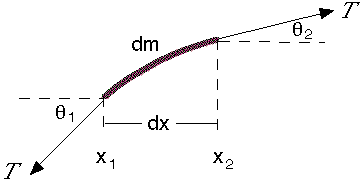# Equations for the velocity of progressive travelling waves

• LCSphysicist
In summary, The classic way of deriving the wave equation for a transverse wave on a tensioned string is to consider a small element and apply Newton's second law in the vertical direction. This results in the wave equation, ##\frac{\partial^2 y}{\partial t^2} = T/μ\frac{\partial^2 y}{\partial x^2}##, where ##T## is the tension and ##μ## is the linear density of the string. This equation allows for traveling wave solutions with a phase velocity of ##v_p = \sqrt{\frac{T}{\mu}} = \frac{\omega}{k}##. Therefore, there is no need for dimensional methods to derive the velocity equation.

#### LCSphysicist

Homework Statement
All below
Relevant Equations
All below
Someone know how to derive v = √(T/μ) for waves traveling? (without being by dimensional analyse)

Look into the wave equation, ##\frac{\partial^2 y}{\partial t^2} = v^2\frac{\partial^2 y}{\partial x^2}##. The classic way of deriving this for a transverse wave on a tensioned string is to consider a small element like thissuch that the tensions on either end are approximately equal, and both angles are small. The linear density of string is ##\mu##, although as it happens it is easier, at least first off, to neglect the contribution of the weight of the element to the resultant force. Try to apply Newton's second law in the vertical direction!

N.B. you might wonder what would happen if we don't neglect the weight. Actually, nothing much, except the wave equation now contains a constant source term.

Last edited by a moderator:
•berkeman
etotheipi said:
Look into the wave equation, ##\frac{\partial^2 y}{\partial t^2} = v^2\frac{\partial^2 y}{\partial x^2}##. The classic way of deriving this for a transverse wave on a tensioned string is to consider a small element like this

View attachment 266517

such that the tensions on either end are approximately equal, and both angles are small. The linear density of string is ##\mu##, although as it happens it is easier, at least first off, to neglect the contribution of the weight of the element to the resultant force. Try to apply Newton's second law in the vertical direction!

N.B. you might wonder what would happen if we don't neglect the weight. Actually, nothing much, except the wave equation now contains a constant source term.

I see what you want yo say, we will get:
##\frac{\partial^2 y}{\partial t^2} = T/μ\frac{\partial^2 y}{\partial x^2}##

The problem is exactly this, we just pick T/μ and assume as the ##v^2##

We just assume it by the dimensional analyse

Okay, let's run it through first, $$T\sin{\theta_2} - T\sin{\theta_1} \approx T\left[ \tan{\theta_2} - \tan{\theta_1} \right] = (\mu dx) \frac{\partial^2 y}{\partial t^2}$$Since ##\tan{\theta} = \frac{\partial y}{\partial x}##,$$T[\tan{\theta_2} - \tan{\theta_1}] = T\left[\frac{\partial^2 y}{\partial x^2} dx \right] = \mu dx \frac{\partial^2 y}{\partial t^2}$$ $$\frac{\partial^2 y}{\partial t^2} = \frac{T}{\mu} \frac{\partial^2 y}{\partial x^2}$$as you inferred. But notice that this differential permits solutions of traveling waves of the form$$y(x,t) = A\sin{(kx - \omega t + \phi)}$$with a phase velocity of ##v_p = \sqrt{\frac{T}{\mu}} = \frac{\omega}{k}##. There is no need for any dimensional methods, or the like!

Last edited by a moderator: